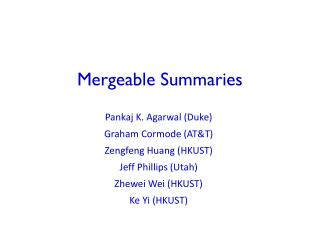DownloadDownload PresentationMergeable Summaries

Mergeable Summaries

Télécharger la présentationMergeable Summaries

- - - - - - - - - - - - - - - - - - - - - - - - - - - E N D - - - - - - - - - - - - - - - - - - - - - - - - - - -
Presentation Transcript

1. Mergeable Summaries Pankaj K. Agarwal (Duke) Graham Cormode (AT&T) Zengfeng Huang (HKUST) Jeff Phillips (Utah) Zhewei Wei (HKUST) Ke Yi (HKUST)

2. Mergeability • Ideally, summaries could behave like simple aggregates: associative, commutative • Oblivious to the computation tree (size and shape) • Error is preserved • Size is bounded • Ideally, independent of base data size • At least sublinear in base data • Rule out “trivial” solution of keeping union of input Mergeable Summaries

3. Models of summary construction • Offline computation: e.g. sort data, take percentiles • Streaming: summary merged with one new item each step • One-time merge: • Equal-weight merges: only merge summaries of same weight • (Full) mergeability: allow arbitrary merging schemes • Data aggregation in sensor networks • Large-scale distributed computation (MUD, MapReduce) Mergeable Summaries

4. Merging: sketches • Example: most sketches (random projections) are mergeable • Count-Min sketch of vector x[1..U]: • Creates a small summary as an array of w  d in size • Use d hash functions h to map vector entries to [1..w] • Estimate x[i] = minj CM[ hj(i), j] • Trivially mergeable: CM(x + y) = CM(x) + CM(y) w Array: CM[i,j] d Mergeable Summaries

5. Summaries for heavy hitters 7 5 1 • Misra-Gries (MG) algorithm finds up to k items that occur more than 1/k fraction of the time in a stream • Keep k different candidates in hand. For each item in stream: • If item is monitored, increase its counter • Else, if < k items monitored, add new item with count 1 • Else, decrease all counts by 1 6 4 2 1 Mergeable Summaries

6. Streaming MG analysis • N = total size of input • M = sum of counters in data structure • Error in any estimated count at most (N-M)/(k+1) • Estimated count a lower bound on true count • Each decrement spread over (k+1) items: 1 new one and k in MG • Equivalent to deleting (k+1) distinct items from stream • At most (N-M)/(k+1) decrement operations • Hence, can have “deleted” (N-M)/(k+1) copies of any item • Set k=1/, the summary estimates any count with error N Mergeable Summaries

7. Merging two MG Summaries • Merging alg: • Merge the counter sets in the obvious way • Take the (k+1)th largest counter = Ck+1, and subtract from all • Delete non-positive counters • Sum of remaining counters is M12 • This alg gives full mergeability: • Merge subtracts at least (k+1)Ck+1from counter sums • So (k+1)Ck+1 (M1 + M2 – M12) • By induction, error is ((N1-M1) + (N2-M2) + (M1+M2–M12))/(k+1)=((N1+N2) –M12)/(k+1) Mergeable Summaries

8. -quantiles: classical results • The classical merge-reduce algorithm • Input: two -quantiles of size 1/ • Merge: combine the two -quantiles, resulting in an -quantiles summary of size 2/ • Reduce: take every other element, size getting down to 1/, error increasing to 2 (the -approximation of an -approximation is a (2)-approximation) • Can also be used to construct -approximations in higher dimensions • Error proportional to the number of levels of merges • Final error is  log n, rescale  to get it right • This is not mergeable! Mergeable Summaries

9. -quantiles: new results • The classical merge-reduce algorithm • Input: two -quantiles of size 1/ • Merge: combine the two -quantiles, resulting in an -quantiles summary of size 2/ • Reduce: pair up consecutive elements and randomly take one, size getting down to 1/, error stays at! • Summary size = O(1/ log1/2 1/) gives an -quantiles summary w/ constant probability • Works only for same-weight merges • The same randomized merging algorithm has been suggested (e.g., Suri et al. 2004), but previous analysis showed that error could still increase Mergeable Summaries

10. Unequal-weight merges • Use equal-size merging in a standard logarithmic trick: • Merge two summaries as binary addition • Fully mergeable quantiles, in O(1/ log n log1/2 1/) • n = number of items summarized, not known a priori • But can we do better? Wt 32 Wt 16 Wt 8 Wt 4 Wt 2 Wt 1 Mergeable Summaries

11. Hybrid summary • Observation: when summary has high weight, low order blocks don’t contribute much • Can’t ignore them entirely, might merge with many small sets • Hybrid structure: • Keep top O(log 1/) levels as before • Also keep a “buffer” sample of (few) items • Merge/keep equal-size summaries, and sample rest into buffer • Analysis rather delicate: • Points go into/out of buffer, but always moving “up” • Size O(1/ log1.5(1/)) gives a correct summary w/ constant prob. Wt 32 Wt 16 Wt 8 Buffer Mergeable Summaries

12. Other mergeable summaries • -approximations in constant VC-dimension d of sizeO(-2d/(d+1) log 2d/(d+1)+1(1/)) • -kernels in d-dimensional space in O((1-d)/2) • For “fat” point sets: bounded ratio between extents in any direction Mergeable Summaries

13. Open Problems • Better bounds than O(1/ log1.5(1/)) for 1D? • Deterministic mergeable -approximations? • Heavy hitters are deterministically mergeable (0 dim) • dim ≥ 1 ? • -kernels without the fatness assumption? • -nets? • Random sample of size O(1/ log(1/)) suffices • Smaller sizes (e.g., for rectangles) • Deterministic? Mergeable Summaries